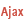Home |Sign in | EnglishHelpWLanguageWLanguage functionsControls, pages and windowsDrawing functionsPrefix syntaxDrawing functions on picLayer variablesDrawSlicePresentationExampleCoordinatesNon-solid figureDrawing with opacity or anti-aliasingPattern and PHPDrawing in Browser codeRelated examplesSee alsoAlphaBlendBackgroundChangeModeDrawChordDrawLineDrawPointDrawPolygonDrawPolylineDrawRectangleDrawRectangleGradientDrawRoundedRectangleDrawSliceDrawTextDrawTextRTFEndDrawingFillFontHorizontalSymmetryInvertColorPenPixelColorPixelOpacityResizeRotationStartDrawingVerticalSymmetry
• Coordinates
• Non-solid figure
• Drawing with opacity or anti-aliasing
• Pattern and PHP
• Drawing in Browser code
WINDEVWEBDEVWINDEV MobileOthers<picLayer variable>.DrawSlice (Function)
In french: <Variable picCalque>.DessinePortion
Draws a portion of a circle or ellipse in an Variable type picLayer.Versions 15 and laterThis function is now available for WEBDEV sites in Linux.New in version 15This function is now available for WEBDEV sites in Linux.This function is now available for WEBDEV sites in Linux.
Example
MyWDPicImage is WDPic = "Test.gif"
MyLayer is picLayer

// Draw a circle section whose
// background is light yellow and whose border is light blue
MyLayer.DrawSlice(10, 10, 50, 50, 20, 20, 40, 50, LightYellow, LightBlue)
IMG_MyDrawing = MyWDPicImage
Syntax
<picLayer image>.DrawSlice(<X1> , <Y1> , <X2> , <Y2> , <X3> , <Y3> , <X4> , <Y4> [, <Background color> [, <Line color>]])
<picLayer image>: picLayer variable
Name of the picLayer variable to use.
<X1>: Integer
X coordinate of top left corner of the rectangle containing the circle. These coordinates are expressed in pixels.
<Y1>: Integer
Y coordinate of top left corner of the rectangle containing the circle. These coordinates are expressed in pixels.
<X2>: Integer
X coordinate of bottom right corner of the rectangle containing the circle. These coordinates are expressed in pixels.
<Y2>: Integer
Y coordinate of bottom right corner of the rectangle containing the circle. These coordinates are expressed in pixels.
<X3>: Integer
X coordinate of start point of the circle section. These coordinates are expressed in pixels.
<Y3>: Integer
Y coordinate of start point of the circle section. These coordinates are expressed in pixels.
<X4>: Integer
X coordinate of end point of the circle section. These coordinates are expressed in pixels.
<Y4>: Integer
Y coordinate of end point of the circle section. These coordinates are expressed in pixels.
<Background color>: Integer or constant (optional)
Background color of circle section. This color can correspond to:
• an RGB color (returned by RGB),
• an HSL color (returned by HSL),
• une preset color of WLanguage,
•Versions 22 and lateran Color variable.New in version 22an Color variable.an Color variable.
If this parameter is not specified, the background color:
<Line color>: Integer or constant (optional)
Color of the line for the circle section. This color can correspond to:
• an RGB color (returned by RGB),
• an HSL color (returned by HSL),
• a preset color of WLanguage,
•Versions 22 and lateran Color variable.New in version 22an Color variable.an Color variable.
If this parameter is not specified, the line color:
• corresponds to the color specified in the last call to <Image>.Pen,
• is identical to the Color used for the Background if the <Image>.Pen function has not been used..
Remarks

Coordinates

If the start point and the end point are not located on the border of the circle (or on the border of the ellipse), the point taken into account corresponds to the intersection between the circle and the line that joins the specified point and the center of the rectangle (which means the center of the circle).Coordinates are given with respect to the top left corner of the image (coordinates: (0.0)).
The drawing sections are drawn anticlockwise.

Non-solid figure

To draw a non-solid figure (without taking into account the center of the circle), use <picLayer variable>.DrawChord.Drawing with opacity or anti-aliasing

In the case of a drawing with opacity (function <picLayer variable>.StartDrawing with the constant dWithOpacity) or anti-aliasing (function <picLayer variable>.ChangeMode with the constant drawAntiAliasing), the contour is drawn by-Above inside the portion: therefore, the line is partly combined with the inside of the section. If the line is very thick, it will be displayed in two colors.
Related Examples:Unit examples (WINDEV): The drawing functions [ + ] Using the main drawing functions of WINDEV to:- Initialize an Image control for drawing- Draw simple shapes- Write a text into a drawing- Change the color in a drawing
Business / UI classification : Neutral code
Component : wd260pnt.dll
Minimum version required
• Version 25